Function Repository Resource:

# WeightedSimpleGraph

SimpleGraph with edge and vertex weights

Contributed by: Nikolay Murzin
 ResourceFunction["WeightedSimpleGraph"][g] gives the underlying simple graph from the graph g with edge weights corresponding to edge multiplicities. ResourceFunction["WeightedSimpleGraph"][g,f] also applies function f to vertices of the graph g and assigns vertex weights based on produced tally.

## Details and Options

ResourceFunction["WeightedSimpleGraph"][g] removes all self-loops and multiple edges between the same vertices.
Self-loops can also be weighted by including SelfLoopsTrue option.
ResourceFunction["WeightedSimpleGraph"] works with undirected graphs, directed graphs, multigraphs, and mixed graphs.

## Examples

### Basic Examples (3)

Remove multi-edges from a graph and assign edge weights with their multiplicities:

 In:=Out=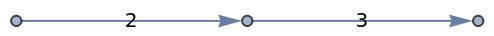Work with mixed graphs:

 In:=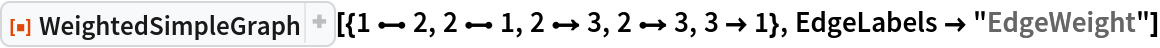Out=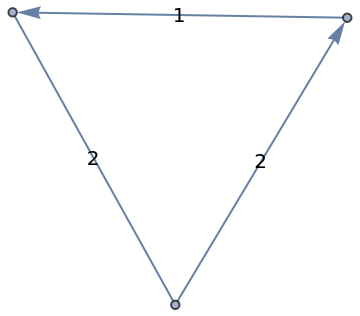Merge each vertex using a function and assign vertex weight based on the number of original vertices producing the same result:

 In:=Out=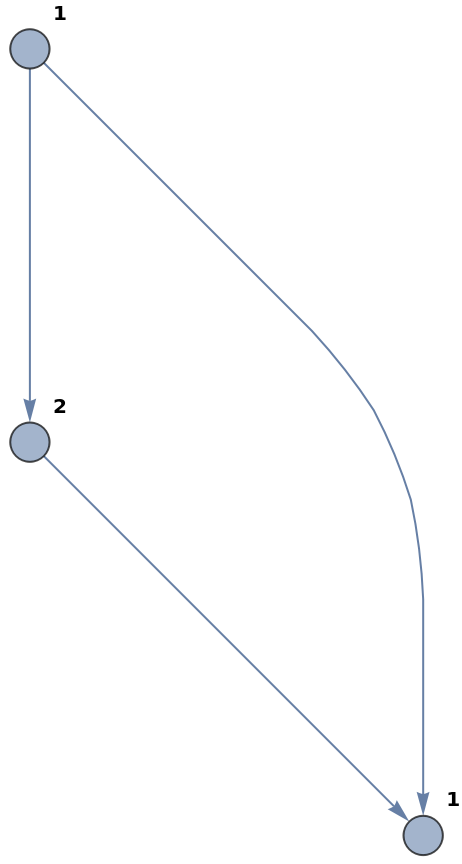### Options (1)

#### SelfLoops (1)

Self-loops can also be weighted:

 In:=Out=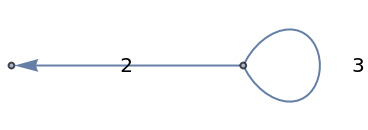### Applications (1)

Produce weighted evolution graph from its full evolution graph for the MultiwaySystem:

 In:=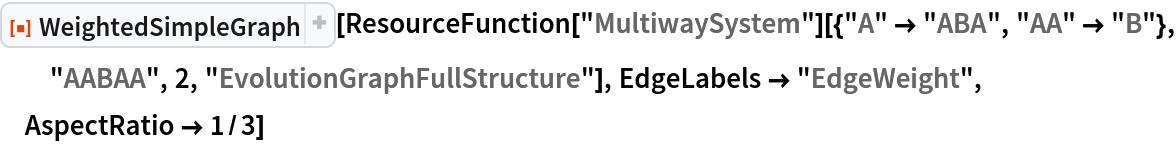Out=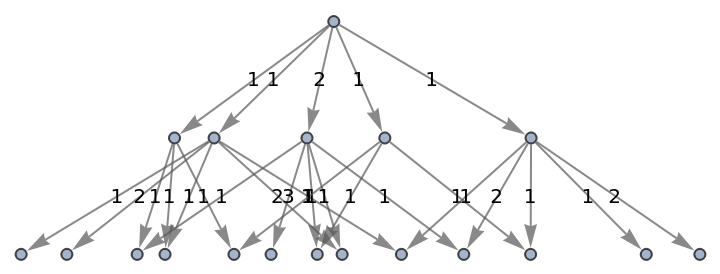In:=Out=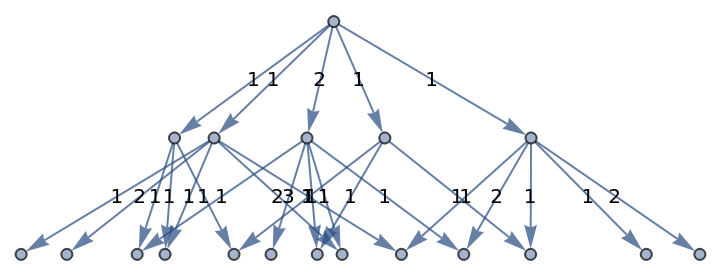### Properties and Relations (1)

Edge weights assigned by WeightedSimpleGraph can also be computed with EdgeMultiplicity:

 In:=Out=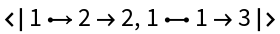## Version History

• 1.1.0 – 03 February 2023
• 1.0.0 – 24 October 2022